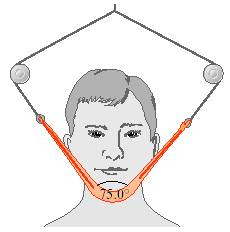# Another Newton's Law of Motion Problem.

• Elkay
In summary, the tension in the strap must be adjusted to produce a net upward force of 5.00 N on the patient's chin.

#### Elkay

Due to a jaw injury, a patient must wear a strap (see the figure) that produces a net upward force of 5.00 N on his chin. The tension is the same throughout the strap. To what tension must the strap be adjusted to provide the necessary upward force? Here's the picture:I think the tension for both sides of the strap will be equal, and the sum of all forces should equal zero. I'm also thinking that the sum of all forces should be zero if this is at equilibrium.
This is what I've done so far (hopefully my handwriting isn't too bad):

I'm under the impression that this problem should be simple, but I've been looking at it and trying to do different things to it yesterday.

Last edited by a moderator:
That's correct; you can now use the second equation to obtain the tension in the strap (since T1=T2).

Your on the right lines; however, there is no need to consider the weight of the head in this case, we don't actually know the weight of the head, you've just assumed it be the same as the required net upwards force. The important point to remember is the required upward force is provided by the sum of the vertical components of both tensions. Neverless, either way you consider the problem you will obtain the same equations, as you have done correctly above. As you correctly said above, due to the symmetry of the problem, both tensions we be equal (i.e. T1=T2=T). Using this, can you simplify your expression for $\sum F_y$?

Edit: That's twice cristo...

Last edited:
Hmm, if I don't consider the weight, just look at the $\sum F_y$ equation and solve for T1, the answer I got was -5...

Last edited by a moderator:
It is true that you don't need to consider weight here. However, you should consider the required upward force. RE:
Hootenanny said:
The important point to remember is the required upward force is provided by the sum of the vertical components of both tensions.
Hence, you should obtain;

$$\sum F_y = T_1\sin52.5 + T_2\sin52.5 = 5$$

Which, by coincidence is identical to your second equation above.

Thank you so much. I think I got the right answer:

Since T1=T2=T, then:

So I guess the key to solving a problem like this is to recognize that the forces are in equilibrium, and to simplify the equations before attempting to solve. Hopefully my text will have a similar problem so I can practice some more.

Last edited by a moderator:

## 1. What is Newton's Second Law of Motion?

Newton's Second Law of Motion states that the acceleration of an object is directly proportional to the net force acting on the object and inversely proportional to its mass. This can be represented by the equation F=ma, where F is force, m is mass, and a is acceleration.

## 2. How do you solve a problem involving Newton's Second Law of Motion?

To solve a problem involving Newton's Second Law of Motion, you first need to identify the given variables, including the force, mass, and acceleration. Then, use the formula F=ma to calculate the unknown variable. Remember to make sure all units are consistent and to use the correct units in the final answer.

## 3. What is the significance of Newton's Second Law of Motion?

Newton's Second Law of Motion is significant because it explains the relationship between force, mass, and acceleration. It is also a fundamental principle in physics and is essential in understanding and predicting the motion of objects.

## 4. Can you give an example of a problem involving Newton's Second Law of Motion?

One example of a problem involving Newton's Second Law of Motion is a car accelerating from rest. If a car with a mass of 1000 kg experiences a net force of 500 N, what is its acceleration? Using the formula F=ma, we can calculate the acceleration to be 0.5 m/s^2.

## 5. How is Newton's Second Law of Motion related to the other laws of motion?

Newton's Second Law of Motion is related to the other laws of motion in that it builds upon and explains the concepts introduced in Newton's First Law (inertia) and Third Law (action and reaction). Together, these laws provide a comprehensive understanding of the behavior of objects in motion.